# Resources tagged with: Addition & subtraction

Filter by: Content type:
Age range:
Challenge level:

### There are 216 results### Nice or Nasty

##### Age 7 to 14 Challenge Level:

There are nasty versions of this dice game but we'll start with the nice ones...### Wild Jack

##### Age 7 to 11 Challenge Level:

A game for 2 or more players with a pack of cards. Practise your skills of addition, subtraction, multiplication and division to hit the target score.### Nice or Nasty for Two

##### Age 7 to 14 Challenge Level:

Some Games That May Be Nice or Nasty for an adult and child. Use your knowledge of place value to beat your opponent.### One Million to Seven

##### Age 7 to 11 Challenge Level:

Start by putting one million (1 000 000) into the display of your calculator. Can you reduce this to 7 using just the 7 key and add, subtract, multiply, divide and equals as many times as you like?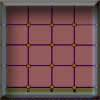### Criss Cross Quiz

##### Age 7 to 11 Challenge Level:

A game for 2 players. Practises subtraction or other maths operations knowledge.### Countdown

##### Age 7 to 14 Challenge Level:

Here is a chance to play a version of the classic Countdown Game.### Napier's Bones

##### Age 7 to 11 Challenge Level:

The Scot, John Napier, invented these strips about 400 years ago to help calculate multiplication and division. Can you work out how to use Napier's bones to find the answer to these multiplications?### Sam's Quick Sum

##### Age 7 to 11 Challenge Level:

What is the sum of all the three digit whole numbers?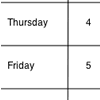### Today's Date - 01/06/2009

##### Age 5 to 11 Challenge Level:

What do you notice about the date 03.06.09? Or 08.01.09? This challenge invites you to investigate some interesting dates yourself.### Scoring with Dice

##### Age 7 to 11 Challenge Level:

I throw three dice and get 5, 3 and 2. Add the scores on the three dice. What do you get? Now multiply the scores. What do you notice?### Number Daisy

##### Age 11 to 14 Challenge Level:

Can you find six numbers to go in the Daisy from which you can make all the numbers from 1 to a number bigger than 25?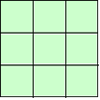### 1, 2, 3 Magic Square

##### Age 7 to 11 Challenge Level:

Arrange three 1s, three 2s and three 3s in this square so that every row, column and diagonal adds to the same total.### Connect Three

##### Age 11 to 16 Challenge Level:

In this game the winner is the first to complete a row of three. Are some squares easier to land on than others?### Cayley

##### Age 11 to 14 Challenge Level:

The letters in the following addition sum represent the digits 1 ... 9. If A=3 and D=2, what number is represented by "CAYLEY"?### Being Resourceful - Primary Number

##### Age 5 to 11 Challenge Level:

Number problems at primary level that require careful consideration.### The Deca Tree

##### Age 7 to 11 Challenge Level:

Find out what a Deca Tree is and then work out how many leaves there will be after the woodcutter has cut off a trunk, a branch, a twig and a leaf.### Two and Two

##### Age 11 to 16 Challenge Level:

How many solutions can you find to this sum? Each of the different letters stands for a different number.### A Square of Numbers

##### Age 7 to 11 Challenge Level:

Can you put the numbers 1 to 8 into the circles so that the four calculations are correct?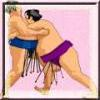### Sumo

##### Age 7 to 11 Challenge Level:

A number game requiring a strategy.### Code Breaker

##### Age 7 to 11 Challenge Level:

This problem is based on a code using two different prime numbers less than 10. You'll need to multiply them together and shift the alphabet forwards by the result. Can you decipher the code?### Domino Numbers

##### Age 7 to 11 Challenge Level:

Can you see why 2 by 2 could be 5? Can you predict what 2 by 10 will be?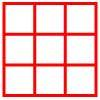##### Age 5 to 11 Challenge Level:

Place six toy ladybirds into the box so that there are two ladybirds in every column and every row.### Diagonal Sums

##### Age 7 to 11 Challenge Level:

In this 100 square, look at the green square which contains the numbers 2, 3, 12 and 13. What is the sum of the numbers that are diagonally opposite each other? What do you notice?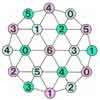### Totality

##### Age 5 to 11 Challenge Level:

This is an adding game for two players.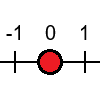### Tug Harder!

##### Age 7 to 11 Challenge Level:

In this game, you can add, subtract, multiply or divide the numbers on the dice. Which will you do so that you get to the end of the number line first?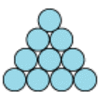### Difference

##### Age 7 to 11 Challenge Level:

Place the numbers 1 to 10 in the circles so that each number is the difference between the two numbers just below it.### Largest Number

##### Age 11 to 14 Challenge Level:

What is the largest number you can make using the three digits 2, 3 and 4 in any way you like, using any operations you like? You can only use each digit once.### Weights

##### Age 11 to 14 Challenge Level:

Different combinations of the weights available allow you to make different totals. Which totals can you make?### Calendar Calculations

##### Age 7 to 11 Challenge Level:

Try adding together the dates of all the days in one week. Now multiply the first date by 7 and add 21. Can you explain what happens?### Got it Article

##### Age 7 to 14

This article gives you a few ideas for understanding the Got It! game and how you might find a winning strategy.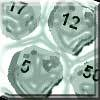### Asteroid Blast

##### Age 7 to 11 Challenge Level:

A game for 2 people. Use your skills of addition, subtraction, multiplication and division to blast the asteroids.### Odds and Threes

##### Age 7 to 11 Challenge Level:

A game for 2 people using a pack of cards Turn over 2 cards and try to make an odd number or a multiple of 3.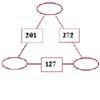### Number Juggle

##### Age 7 to 11 Challenge Level:

Fill in the missing numbers so that adding each pair of corner numbers gives you the number between them (in the box).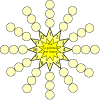### The Twelve Pointed Star Game

##### Age 7 to 11 Challenge Level:

Have a go at this game which involves throwing two dice and adding their totals. Where should you place your counters to be more likely to win?### The Clockmaker's Birthday Cake

##### Age 7 to 11 Challenge Level:

The clockmaker's wife cut up his birthday cake to look like a clock face. Can you work out who received each piece?### The 24 Game

##### Age 7 to 11 Challenge Level:

There are over sixty different ways of making 24 by adding, subtracting, multiplying and dividing all four numbers 4, 6, 6 and 8 (using each number only once). How many can you find?### Pair Sums

##### Age 11 to 14 Challenge Level:

Five numbers added together in pairs produce: 0, 2, 4, 4, 6, 8, 9, 11, 13, 15 What are the five numbers?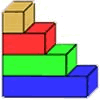### Rod Measures

##### Age 7 to 11 Challenge Level:

Using 3 rods of integer lengths, none longer than 10 units and not using any rod more than once, you can measure all the lengths in whole units from 1 to 10 units. How many ways can you do this?### I'm Eight

##### Age 5 to 11 Challenge Level:

Find a great variety of ways of asking questions which make 8.### Zios and Zepts

##### Age 7 to 11 Challenge Level:

On the planet Vuv there are two sorts of creatures. The Zios have 3 legs and the Zepts have 7 legs. The great planetary explorer Nico counted 52 legs. How many Zios and how many Zepts were there?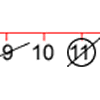### Strike it Out for Two

##### Age 5 to 11 Challenge Level:

Strike it Out game for an adult and child. Can you stop your partner from being able to go?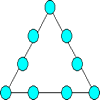### Magic Triangle

##### Age 7 to 11 Challenge Level:

Place the digits 1 to 9 into the circles so that each side of the triangle adds to the same total.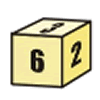### First Connect Three for Two

##### Age 7 to 11 Challenge Level:

First Connect Three game for an adult and child. Use the dice numbers and either addition or subtraction to get three numbers in a straight line.### Reverse Trick

##### Age 7 to 11 Challenge Level:

Tell your friends that you have a strange calculator that turns numbers backwards. What secret number do you have to enter to make 141 414 turn around?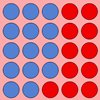### Place Value as a Building Block for Developing Fluency in the Calculation Process

##### Age 5 to 11

This article for primary teachers encourages exploration of two fundamental ideas, exchange and 'unitising', which will help children become more fluent when calculating.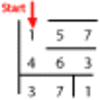### Maze 100

##### Age 7 to 11 Challenge Level:

Can you go through this maze so that the numbers you pass add to exactly 100?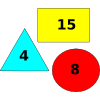### Super Value Shapes

##### Age 7 to 11 Challenge Level:

If each of these three shapes has a value, can you find the totals of the combinations? Perhaps you can use the shapes to make the given totals?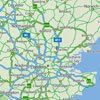### Journeys

##### Age 7 to 11 Challenge Level:

Investigate the different distances of these car journeys and find out how long they take.### First Connect Three

##### Age 7 to 14 Challenge Level:

Add or subtract the two numbers on the spinners and try to complete a row of three. Are there some numbers that are good to aim for?### Making Sense of Positives and Negatives

##### Age 11 to 14

This article suggests some ways of making sense of calculations involving positive and negative numbers.